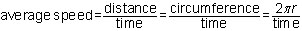# Circular Motion Problems

You can determine the average speed of an object in UCM in a similar way that you did for an object traveling in a straight line.The distance of one complete cycle around a circle is known as the circumference. It can be calculated by multiplying 2π (π equals 3.14) times by the radius of the circular path.